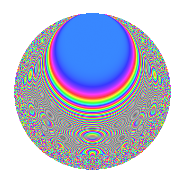# Properties

 Label 320.6.lLevel 320 Weight 6 Character orbit l Rep. character $$\chi_{320}(81,\cdot)$$ Character field $$\Q(\zeta_{4})$$ Dimension 80 Newform subspaces 1 Sturm bound 288 Trace bound 0

# Related objects

## Defining parameters

 Level: $$N$$ $$=$$ $$320 = 2^{6} \cdot 5$$ Weight: $$k$$ $$=$$ $$6$$ Character orbit: $$[\chi]$$ $$=$$ 320.l (of order $$4$$ and degree $$2$$) Character conductor: $$\operatorname{cond}(\chi)$$ $$=$$ $$16$$ Character field: $$\Q(i)$$ Newform subspaces: $$1$$ Sturm bound: $$288$$ Trace bound: $$0$$

## Dimensions

The following table gives the dimensions of various subspaces of $$M_{6}(320, [\chi])$$.

Total New Old
Modular forms 496 80 416
Cusp forms 464 80 384
Eisenstein series 32 0 32

## Trace form

 $$80q + O(q^{10})$$ $$80q - 1208q^{11} + 1800q^{15} - 2360q^{19} + 7464q^{27} - 8144q^{29} + 21296q^{37} - 32072q^{43} + 88360q^{47} - 192080q^{49} + 5920q^{51} - 49456q^{53} - 44984q^{59} + 48080q^{61} - 158760q^{63} - 61160q^{67} - 22320q^{69} - 14896q^{77} - 177680q^{79} - 524880q^{81} + 329240q^{83} + 132400q^{85} - 364832q^{91} - 362352q^{93} - 288800q^{95} - 659000q^{99} + O(q^{100})$$

## Decomposition of $$S_{6}^{\mathrm{new}}(320, [\chi])$$ into newform subspaces

Label Dim. $$A$$ Field CM Traces $q$-expansion
$$a_2$$ $$a_3$$ $$a_5$$ $$a_7$$
320.6.l.a $$80$$ $$51.323$$ None $$0$$ $$0$$ $$0$$ $$0$$

## Decomposition of $$S_{6}^{\mathrm{old}}(320, [\chi])$$ into lower level spaces

$$S_{6}^{\mathrm{old}}(320, [\chi]) \cong$$ $$S_{6}^{\mathrm{new}}(16, [\chi])$$$$^{\oplus 6}$$$$\oplus$$$$S_{6}^{\mathrm{new}}(64, [\chi])$$$$^{\oplus 2}$$$$\oplus$$$$S_{6}^{\mathrm{new}}(80, [\chi])$$$$^{\oplus 3}$$

## Hecke characteristic polynomials

There are no characteristic polynomials of Hecke operators in the database